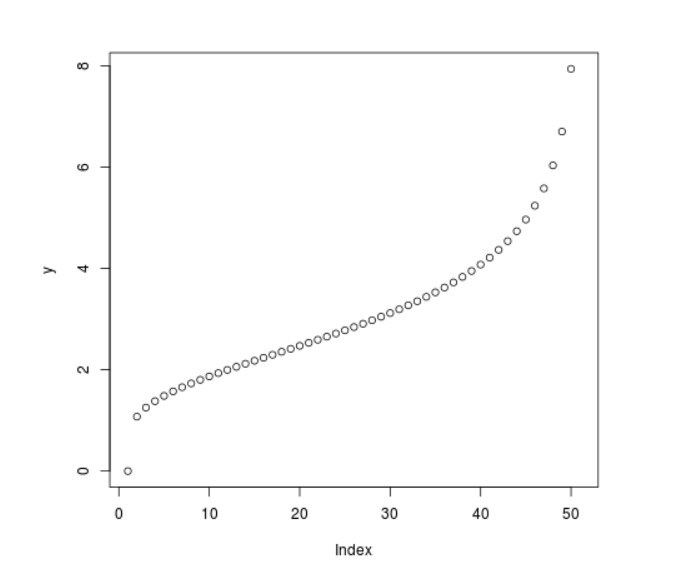Compute the value of Quantile Function over Studentized Distribution in R Programming – qtukey() Function

• Last Updated : 25 Jun, 2020

qtukey() function in R Language is used to compute the value of Studentized Range Quantile Function over a sequence of Numeric Values.

Syntax: qtukey(x, nmeans, df)

Parameters:
x: Numeric Vector
nmeans: Number of means
df: Degree of Freedom

Example 1:

 # R Program to compute the value of# Quantile Function Studentized Range  # Creating a sequence of Numeric valuesx <- seq(0, 1, by = 0.1)  # Calling qtukey() Functiony <- qtukey(x, nmeans = 7, df = 5)y

Output:

 0.000000 1.574255 1.933986 2.237255 2.531898 2.842588 3.193850 3.623645
 4.214094 5.238196      Inf

Example 2:

 # R Program to compute the value of# Quantile Function Studentized Range  # Creating a sequence of Numeric valuesx <- seq(0, 1, by = 0.02)  # Calling qtukey() Functiony <- qtukey(x, nmeans = 7, df = 5)  # Plot a graphplot(y)

Output:My Personal Notes arrow_drop_up